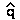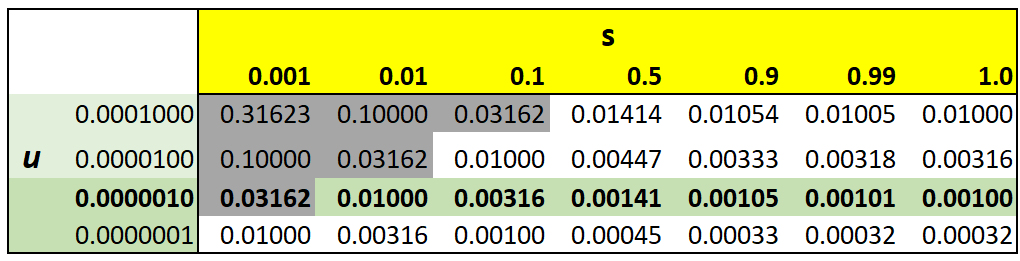Mutation / selection equilibrium

Deleterious alleles maintained by recurrent mutation.
stable equilibrium(read as "q hat," where= 0) reached
when rate of replacement (by mutation)
balances rate of removal (by selection).

µ = frequency of new mutant alleles per locus per generation
µ = 10-6 : 1 in 1,000,000 gametes has new mutant

then=  $\sqrt\left\{\cdots \right\}$    [see derivation below]

Ex.: For recessive lethal allele (= 1) with mutation rate of µ = 10-6
then=   $\sqrt\left\{\cdots \right\}$   =    $\sqrt\left\{\cdots \right\}$0.001

Lowered selection against deleterious allele increases frequency
Medical intervention increases frequency of heritable conditions
in Homo (e.g., diabetes, myopia)
Eugenics: 1920s ~ 1960s social policy
Modification of human condition by selective breeding

'positive eugenics': encouraging people with "good genes" to breed
'negative eugenics': discouraging people with "bad genes'' from breeding
e.g., immigration control, compulsory sterilization, and worse
[See: SJ Gould, "The Mismeasure of Man"]

Is eugenics effective at reducing frequency of deleterious alleles?
What proportion of 'deleterious alleles' found in heterozygous carriers?

(2pq) / 2q2 = p/q1/q    (if  q << 1)

for s = 1, ratio 1000 / 1 : most variant alleles in heterozygotes,
not subject to selection

Conclusion: if most mutations are rare (u < 0.001) & selectively disadvantageous ( s > 0.01)
mutation will not maintain population variation at high levels observed:for paired values of s & µ by approximationHOMEWORK: Suppose myopia is due to a recessive allele B at a single locus, with mutation rate µ = 10-5 from AB.
Suppose that myopia historically resulted in a selection coefficient s = 0.3,
and that vision correction has reduced selection by 90%.
1)
What is the former vs the new equilibrium frequencyof the B allele?
2) What is the former vs the new
equilibrium frequency of persons with myopia ?

Derivation of Mutation / Selection equilibrium

Consider rare, recessive, deleterious allele a

f(a) = q << 1   &   f(A) = p ~ 1
f(Aa) =  µmutation rate (# new mutant alleles / gamete): equilibrium between loss of a due to selection
& replacement of a by new mutation

change in f(a) due to selectionqs = -spq2 / (1 - sq2)     [complete dominance model]
change in f(a) due to mutationqµ  = µp

Thenqµ  +q =  µp -  spq2 / (1 - sq2)µ -  spq                    [ (1 - sq2)1   if   q << p ]
=  (p) (µ - sq2)

At equilibriumq = 0 = (p) (µ - s2)µ - s2          [if1 ]
s2 =  µ2 =  µ / s=µ/s)0.5

Text material © 2021 by Steven M. Carr